# 准备数据

git clone https://github.com/tbragin/elasticon_tour16_demo

tar xzf ncedc-earthquakes-dataset.tar.gz

ncedc-earthquakes-logstash.conf

input {
stdin { type => "earthquake" }
}
filter {
csv {
columns => ["timestamp","latitude","longitude","depth","mag","magType","nst","gap","dmin","rms","source","event_id"]
convert => {"latitude" => "float"}
convert => {"longitude" => "float"}
convert => {"depth" => "float"}
convert => {"mag" => "float"}
convert => {"dmin" => "float"}
convert => {"rms" => "float"}
convert => {"gap" => "float"}
}
mutate {
remove_field => ["latitude", "longitude"]
}
date {
match => ["timestamp", "yyyy/MM/dd HH:mm:ss.SS", "ISO8601"]
remove_field => ["timestamp"]
}
}
output {
stdout { codec => rubydebug { metadata => true } }
stdout { codec => dots }
elasticsearch {
hosts => ["localhost:9200"]
user => "elastic"
index => "ncedc-earthquakes"
template => "ncedc-earthquakes-template.json"
template_name => "ncedc-earthquakes"
template_overwrite => true
}
}

$pwd /Users/liuxg/elastic5/logstash-7.9.1 liuxg:logstash-7.9.1 liuxg$ ls
CONTRIBUTORS                    lib
Gemfile                         logstash-core
Gemfile.lock                    logstash-core-plugin-api
NOTICE.TXT                      ncedc-earthquakes-logstash.conf
bin                             ncedc-earthquakes-template.json
config                          tools
data                            vendor
earthquakes.txt                 x-pack

ncedc-earthquakes-template.json

{
"template": "ncedc-earthquakes",
"settings": {
"number_of_shards": 1,
"number_of_replicas" : 0,
"index.refresh_interval": "60s"
},
"mappings": {
"dynamic_templates": [
{
"strings": {
"match": "*",
"match_mapping_type": "string",
"mapping": {
"type": "text"
}
}
}
],
"properties": {
"location": {
"type": "geo_point"
}
}
}
}

上面的目的就是为了使得我们创建的 location 字段为 geo_point 数据类型。我们通过如下的命令来把数据导入到 Elasticsearch 中：

cat earthquakes.txt| bin/logstash -f ncedc-earthquakes-logstash.conf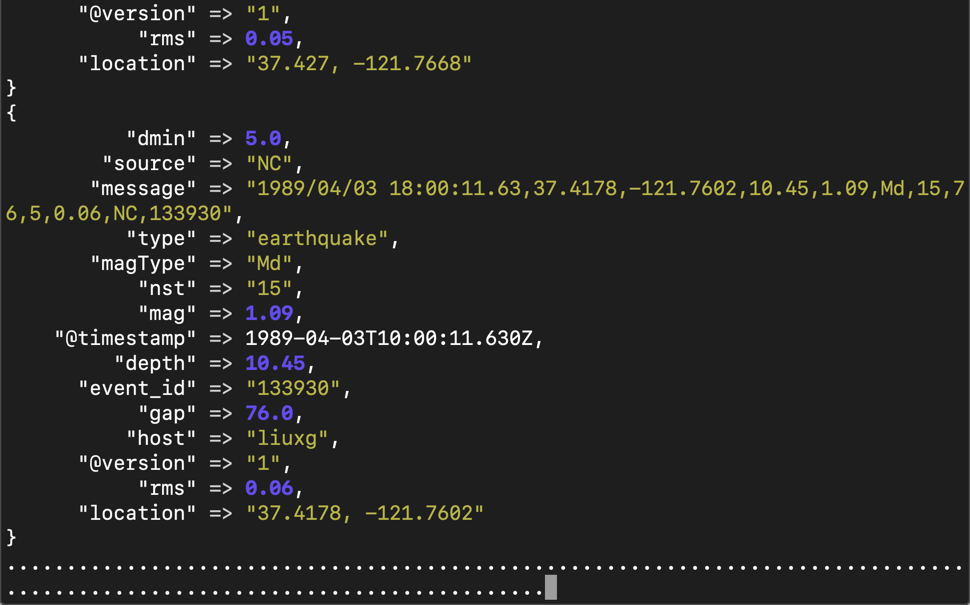GET _cat/indices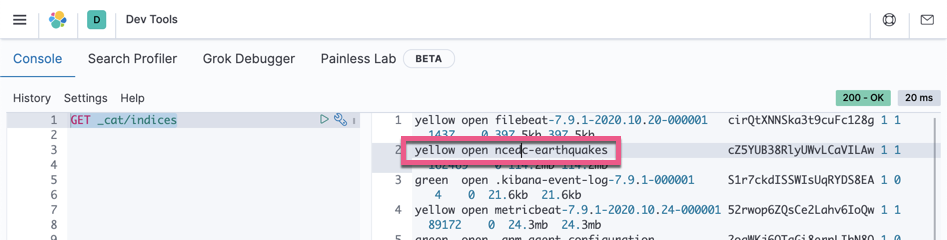GET ncedc-earthquakes/_count
{
"count" : 2394927,
"_shards" : {
"total" : 1,
"successful" : 1,
"skipped" : 0,
"failed" : 0
}
}

{
"message" : "2009/05/13 22:52:58.10,37.8677,-122.2295,11.00,1.35,Md,24,45,1,0.06,NC,51221532",
"gap" : 45.0,
"host" : "liuxg",
"source" : "NC",
"@timestamp" : "2009-05-13T14:52:58.100Z",
"type" : "earthquake",
"magType" : "Md",
"location" : "37.8677, -122.2295",
"dmin" : 1.0,
"depth" : 11.0,
"@version" : "1",
"mag" : 1.35,
"nst" : "24",
"event_id" : "51221532",
"rms" : 0.06
}

# 使用 Timelion 做数据分析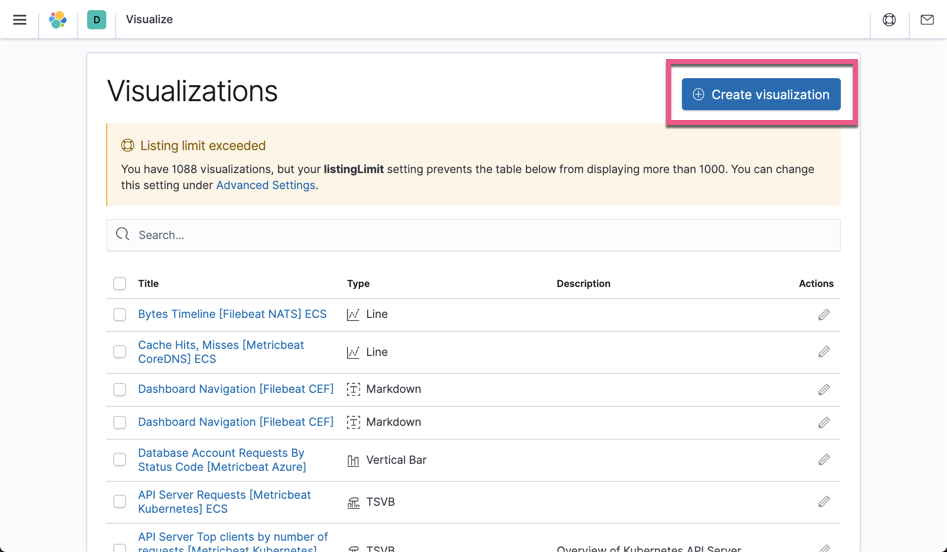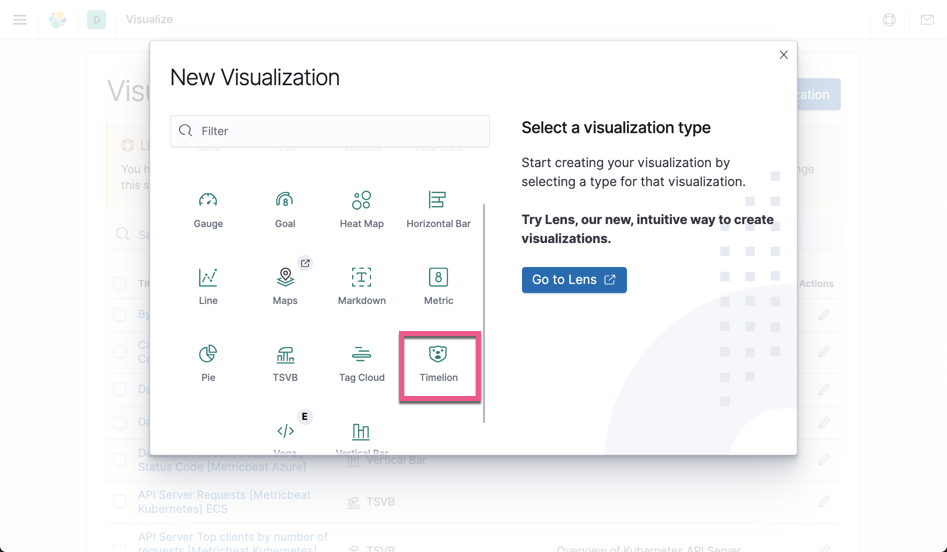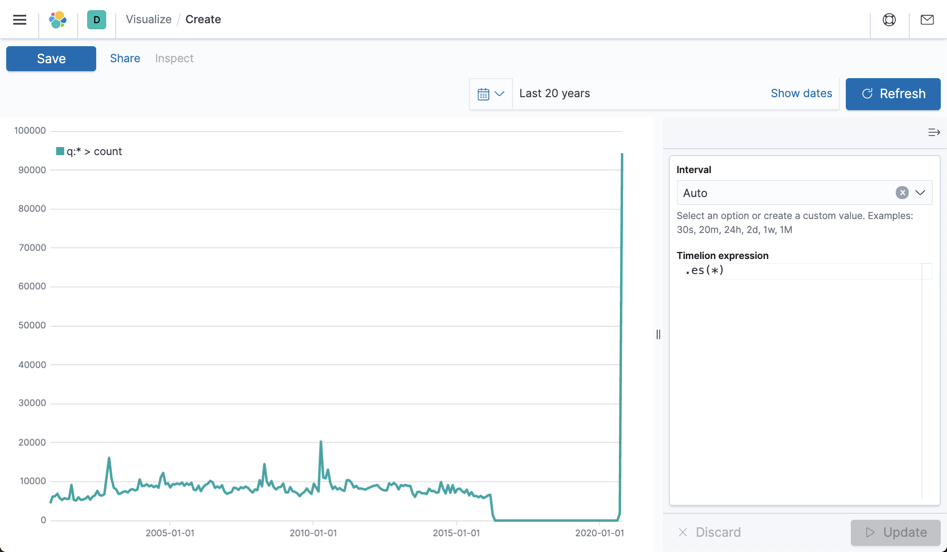.es(index=ncedc-earthquakes,metric=avg:depth).points(fill=5).color(#8cd98c).label('Depth - raw').yaxis(label="Depth"),.es(index=ncedc-earthquakes,metric=avg:depth).lines().movingaverage(52).label('Depth - moving avg').yaxis(label="Depth").color(#257425 ), .es(index=ncedc-earthquakes,metric=avg:mag).points(fill=5).label('Magnitude - raw').yaxis(2, label="Magnitude").color(#ff6666), .es(index=ncedc-earthquakes,metric=avg:mag).lines().movingaverage(52).label('Magnitude - moving avg').yaxis(2, label="Magnitude").color(#b30000 )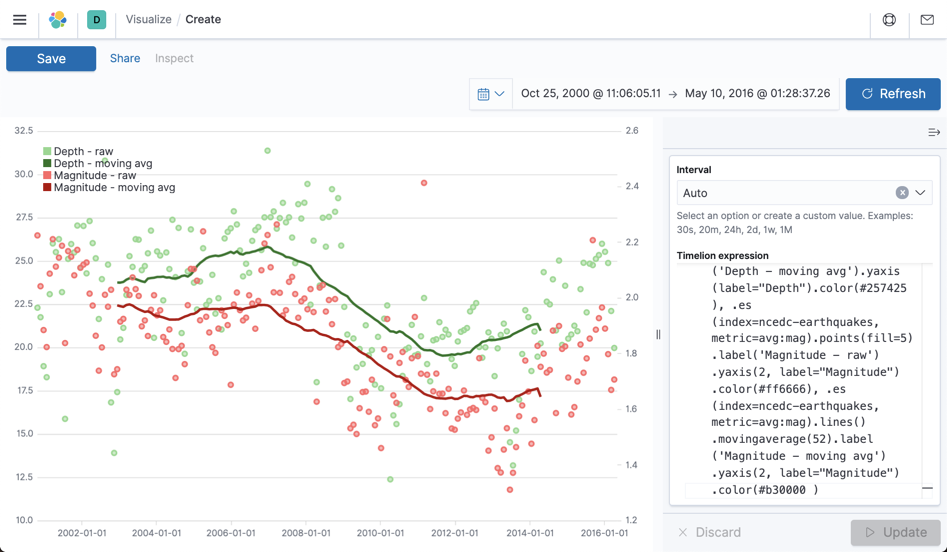.es(index=ncedc-earthquakes,metric=avg:mag).points(fill=5).label('raw').yaxis(2, label="Magnitude").color(#ffa3a3), .es(index=ncedc-earthquakes,metric=avg:mag).lines().movingaverage(52).label('5 year moving avg').yaxis(2, label="Magnitude").color(#b30000 ), .es(index=ncedc-earthquakes,metric=avg:mag).lines().movingaverage(10).label('1 year moving avg').yaxis(2, label="Magnitude").color(#ff3333)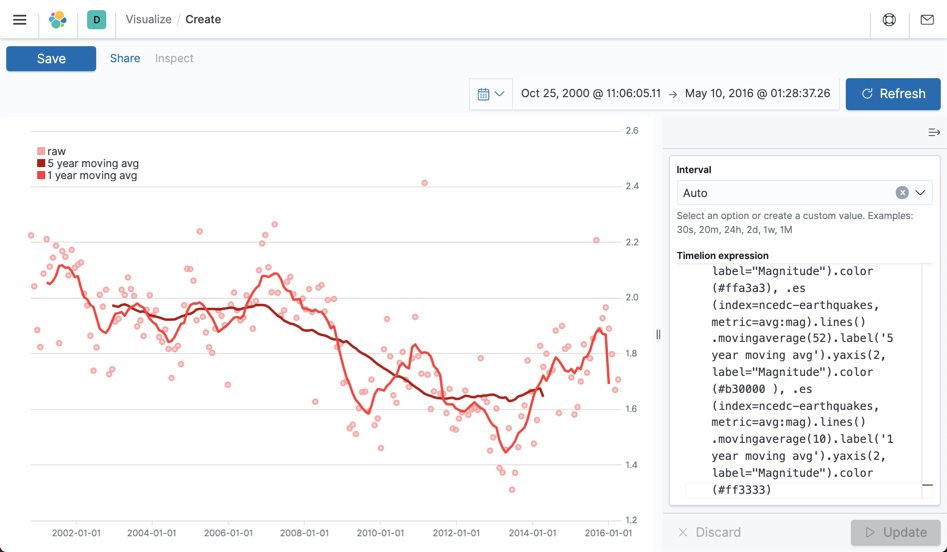.es(index=ncedc-earthquakes,metric=avg:depth).points(fill=5).color(#a3e0a3).label('raw').yaxis(label="Depth"),.es(index=ncedc-earthquakes,metric=avg:depth).lines().movingaverage(52).label('5 year moving avg').yaxis(label="Depth").color(#257425 ), .es(index=ncedc-earthquakes,metric=avg:depth).lines().movingaverage(10).label('1 year moving avg').yaxis(label="Depth").color(#00b359)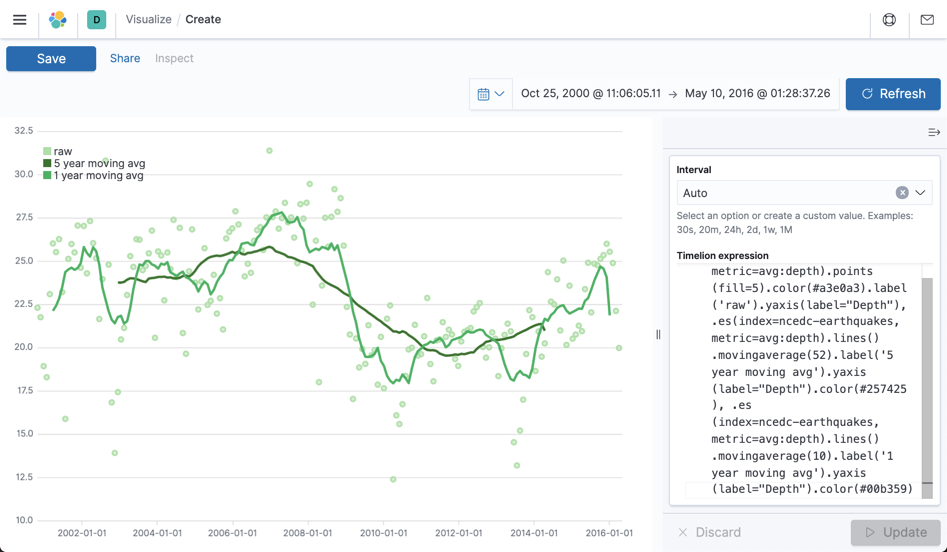# 一步一步建立可视化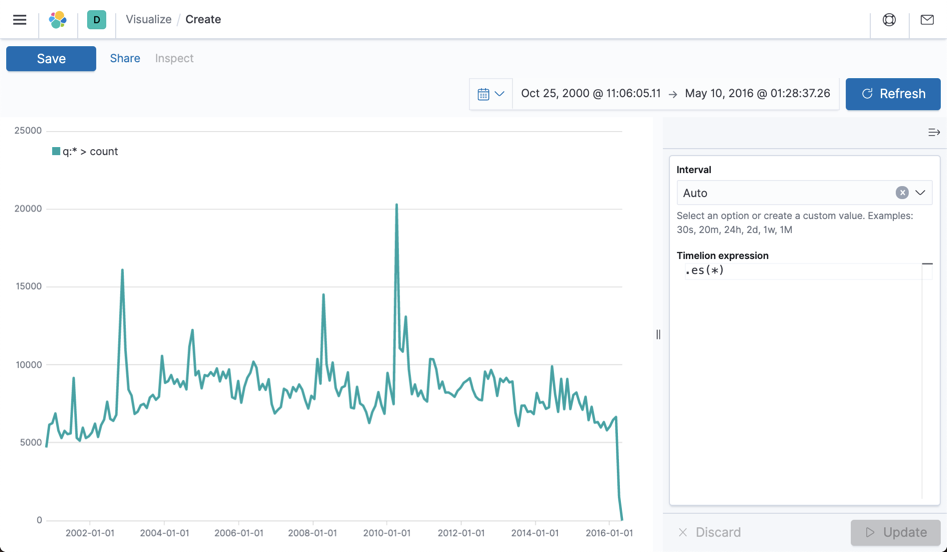.es(index=ncedc-earthquakes,metric=max:mag)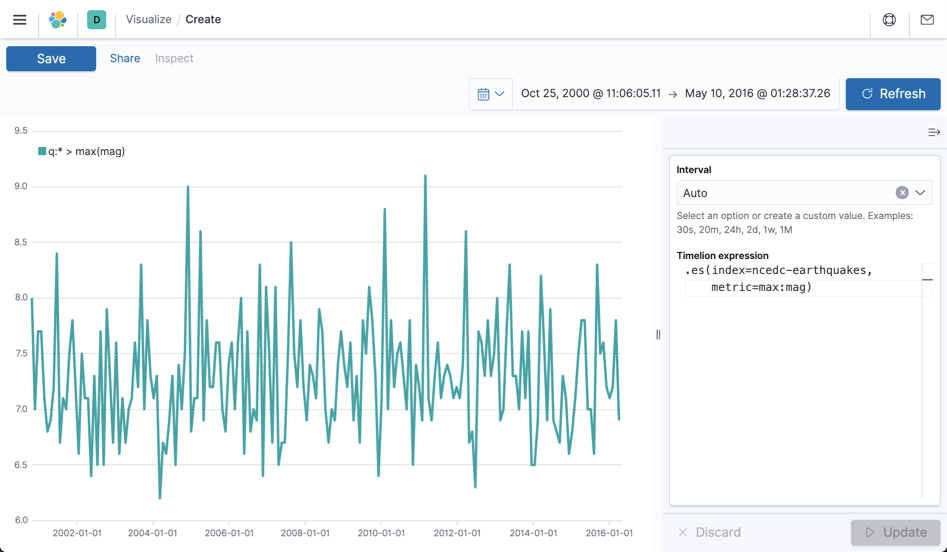.es(index=ncedc-earthquakes,metric=max:mag),.es(index=ncedc-earthquakes,metric=max:mag).mvavg(10)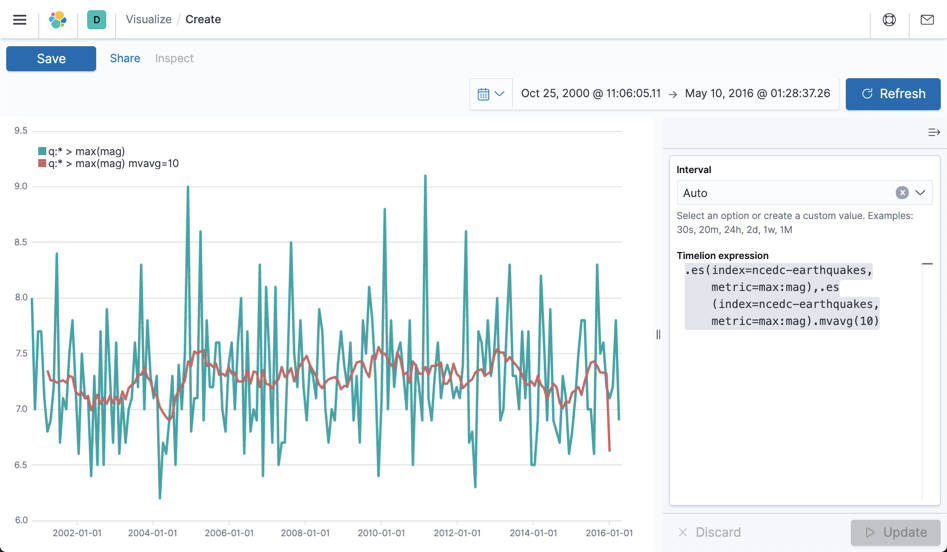.es(index=ncedc-earthquakes,metric=max:mag).points(),.es(index=ncedc-earthquakes,metric=max:mag).mvavg(10)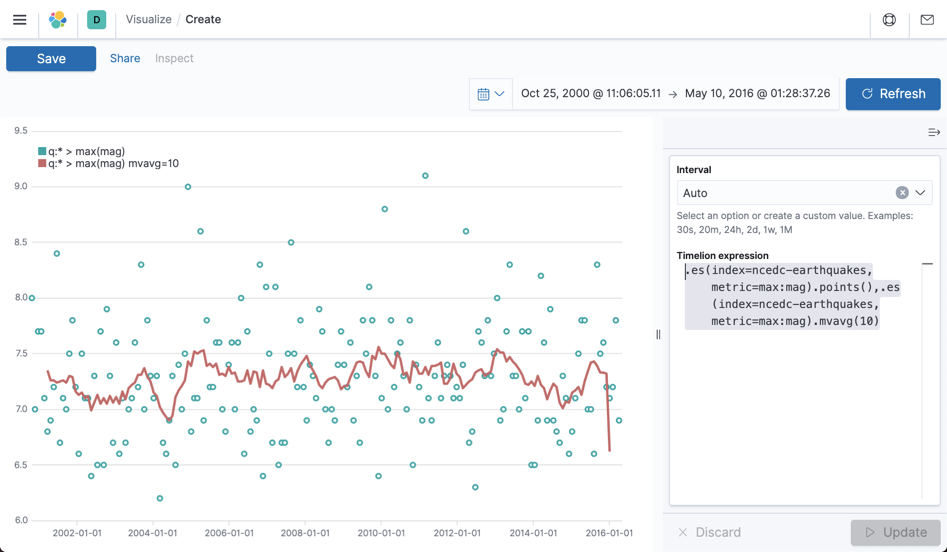.es(index=ncedc-earthquakes,metric=max:mag).points().color(blue),.es(index=ncedc-earthquakes,metric=max:mag).mvavg(10).color(orange)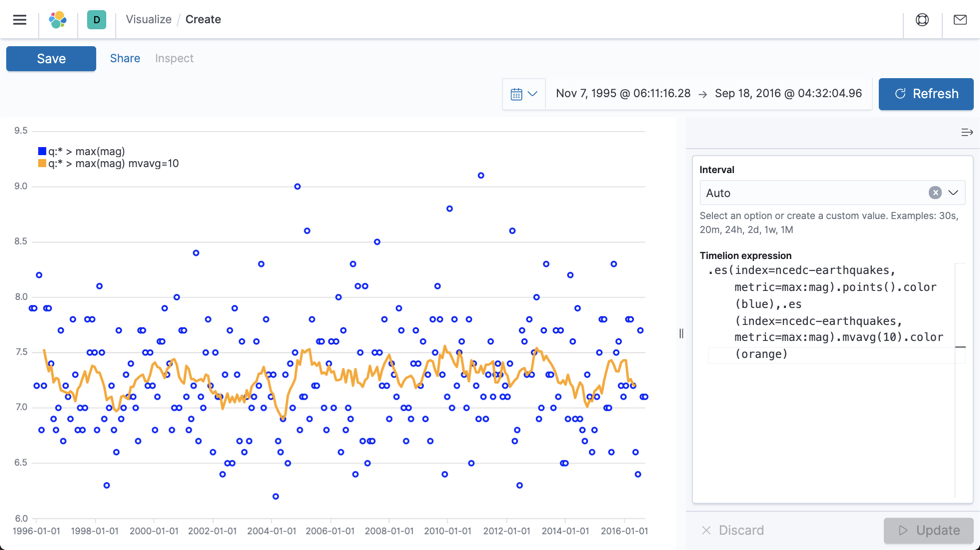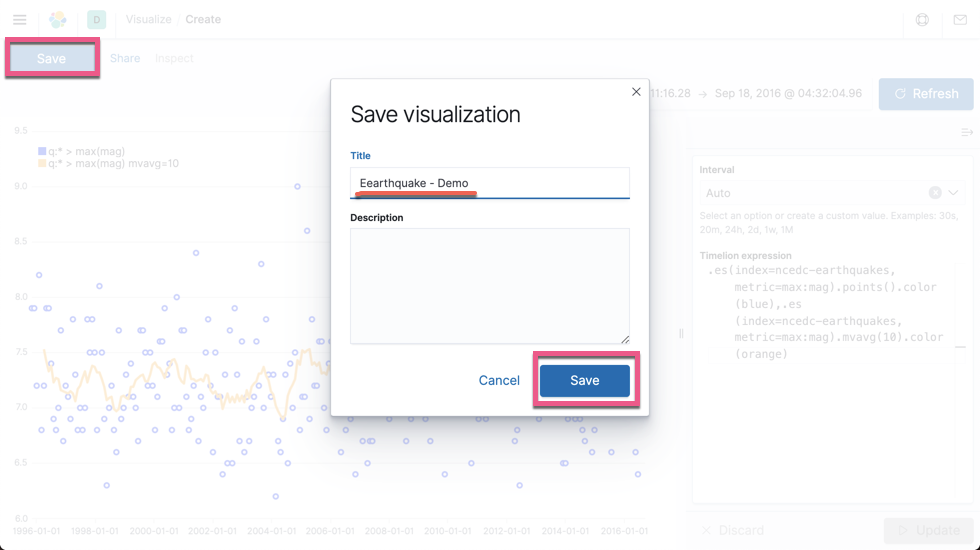# 创建 Maps 可视化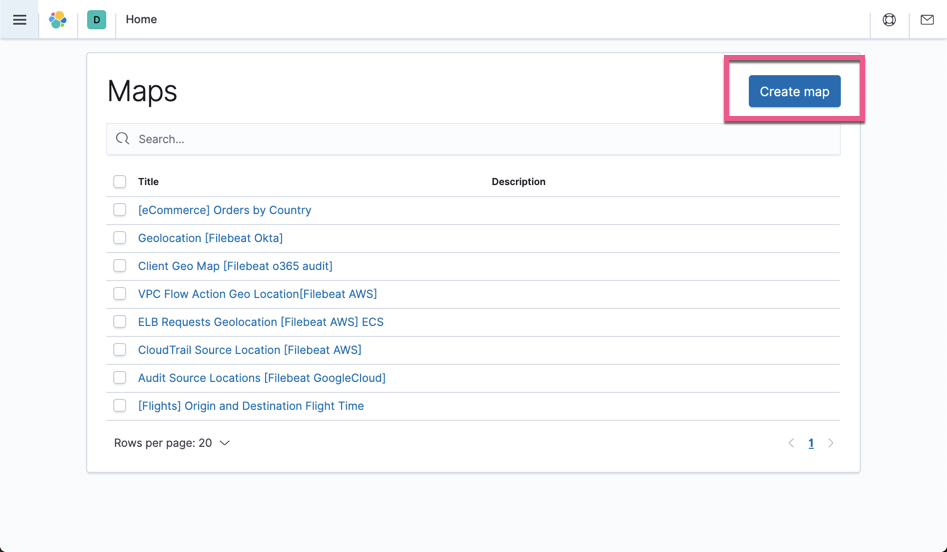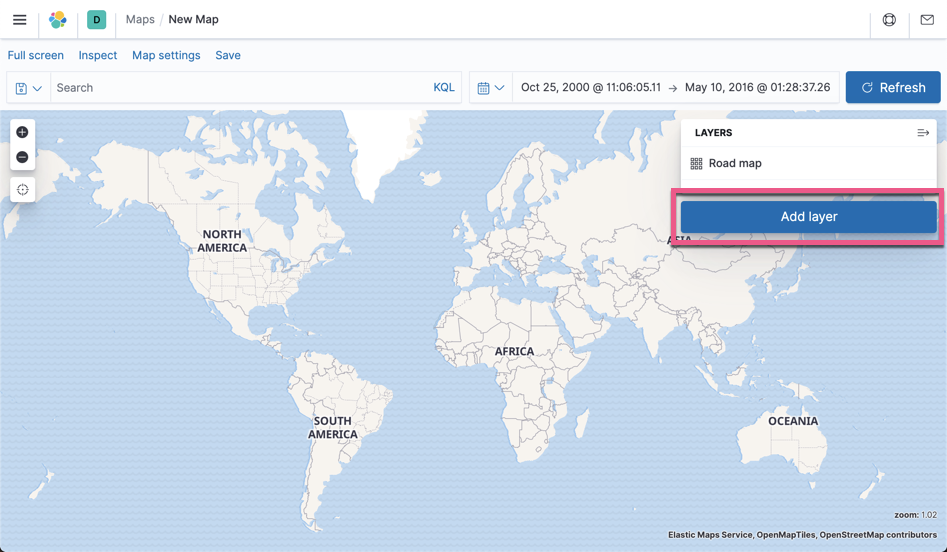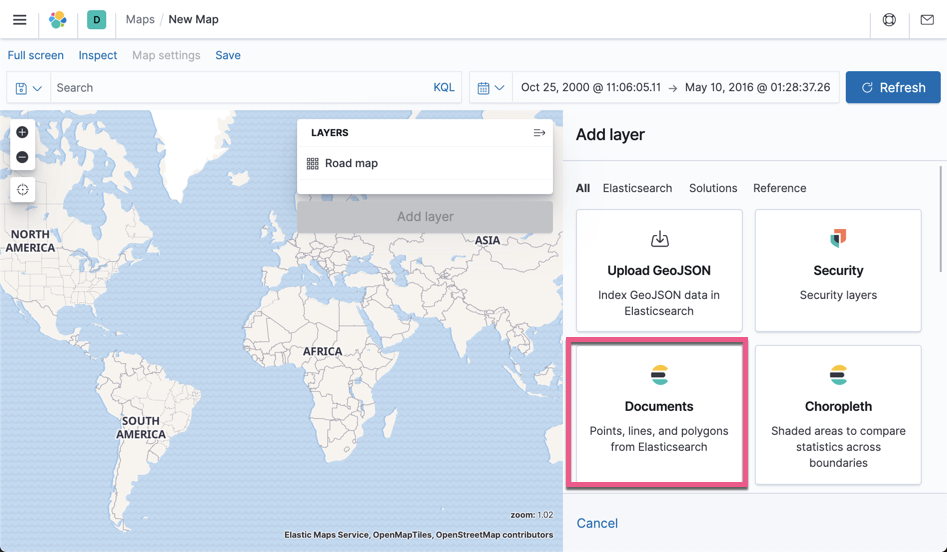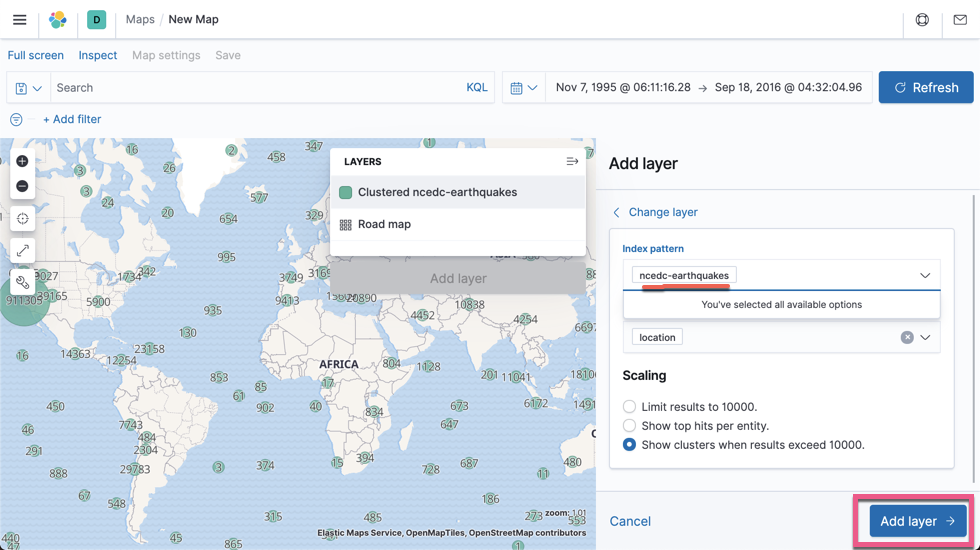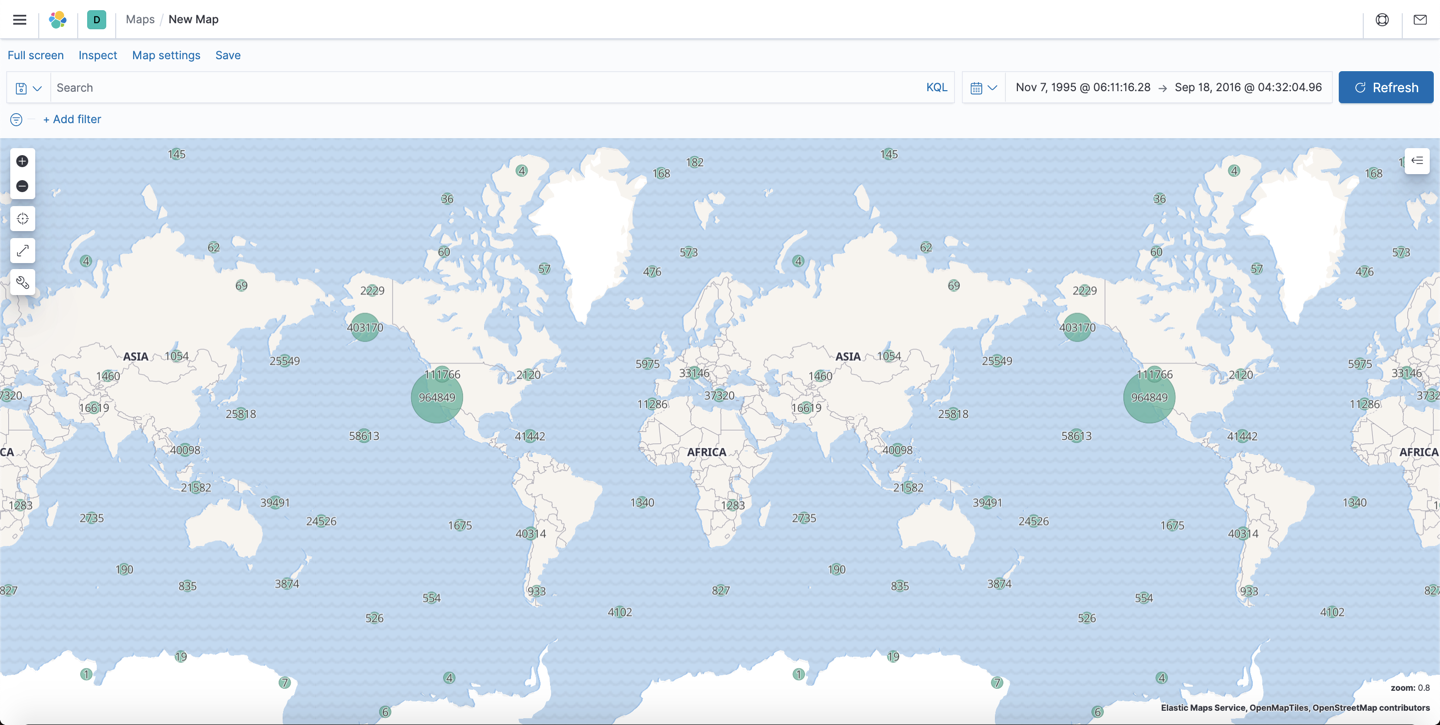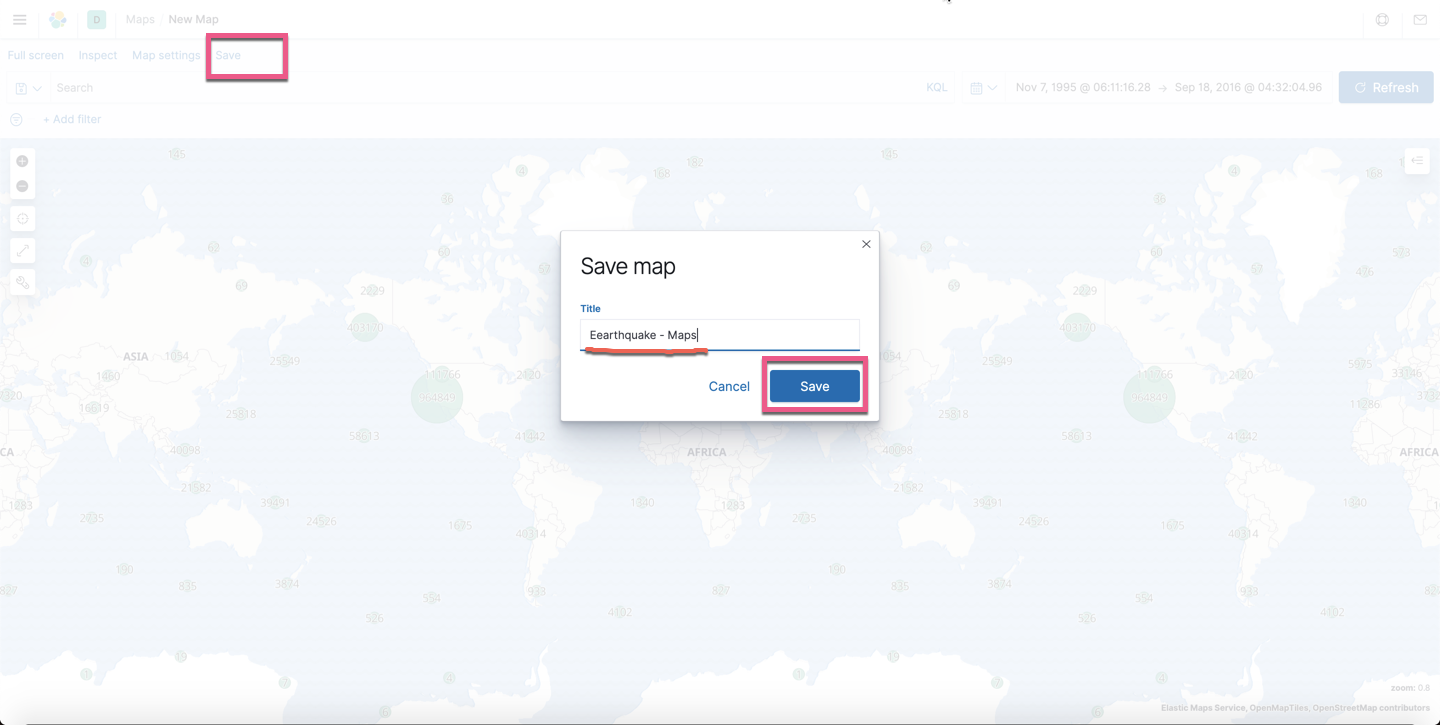# 创建 Eearthquake Dashboard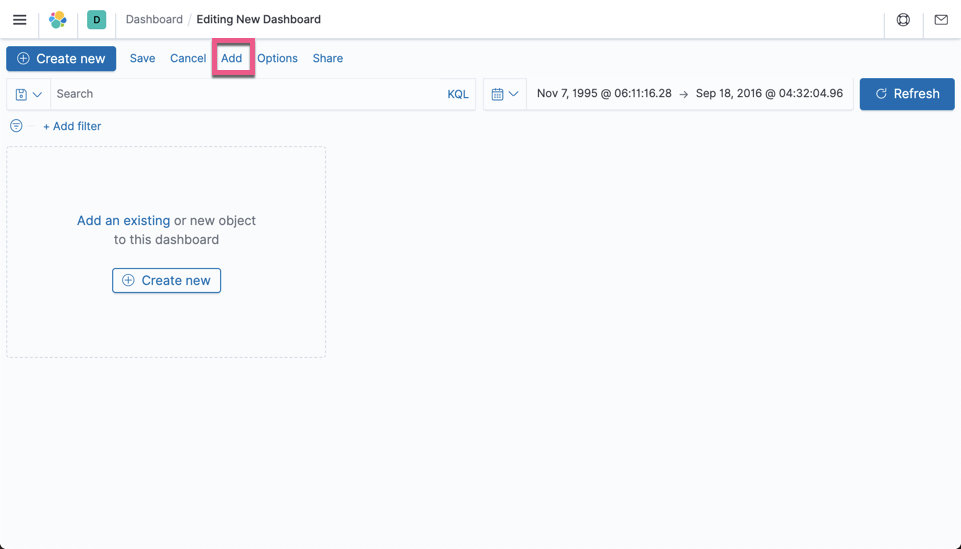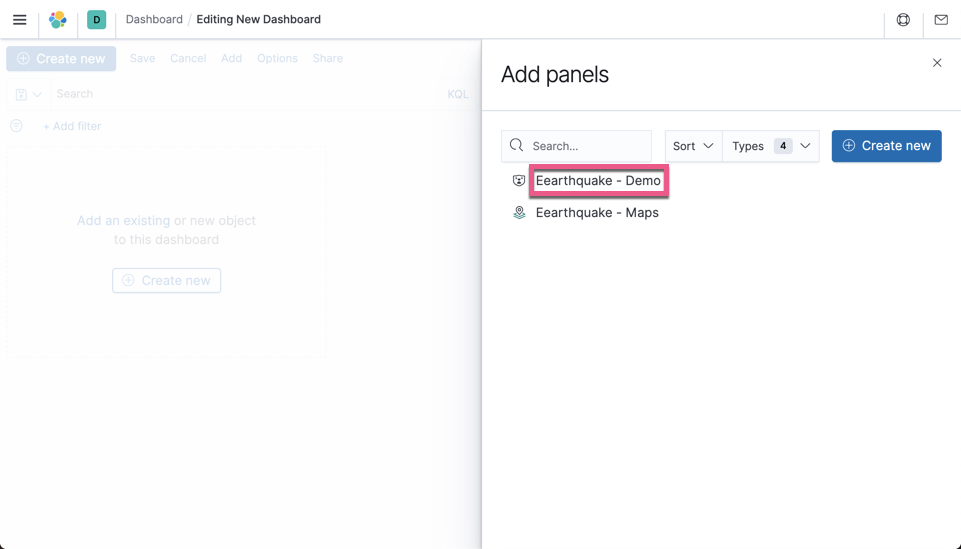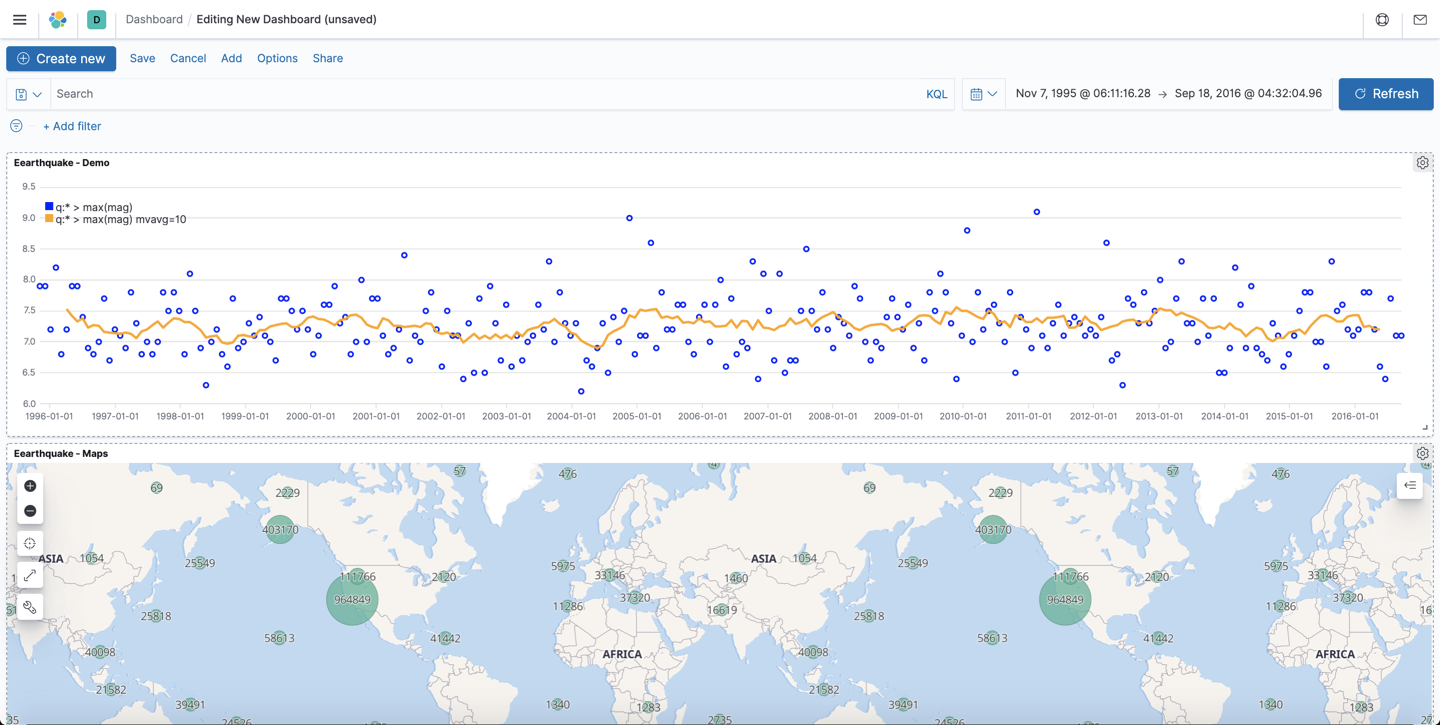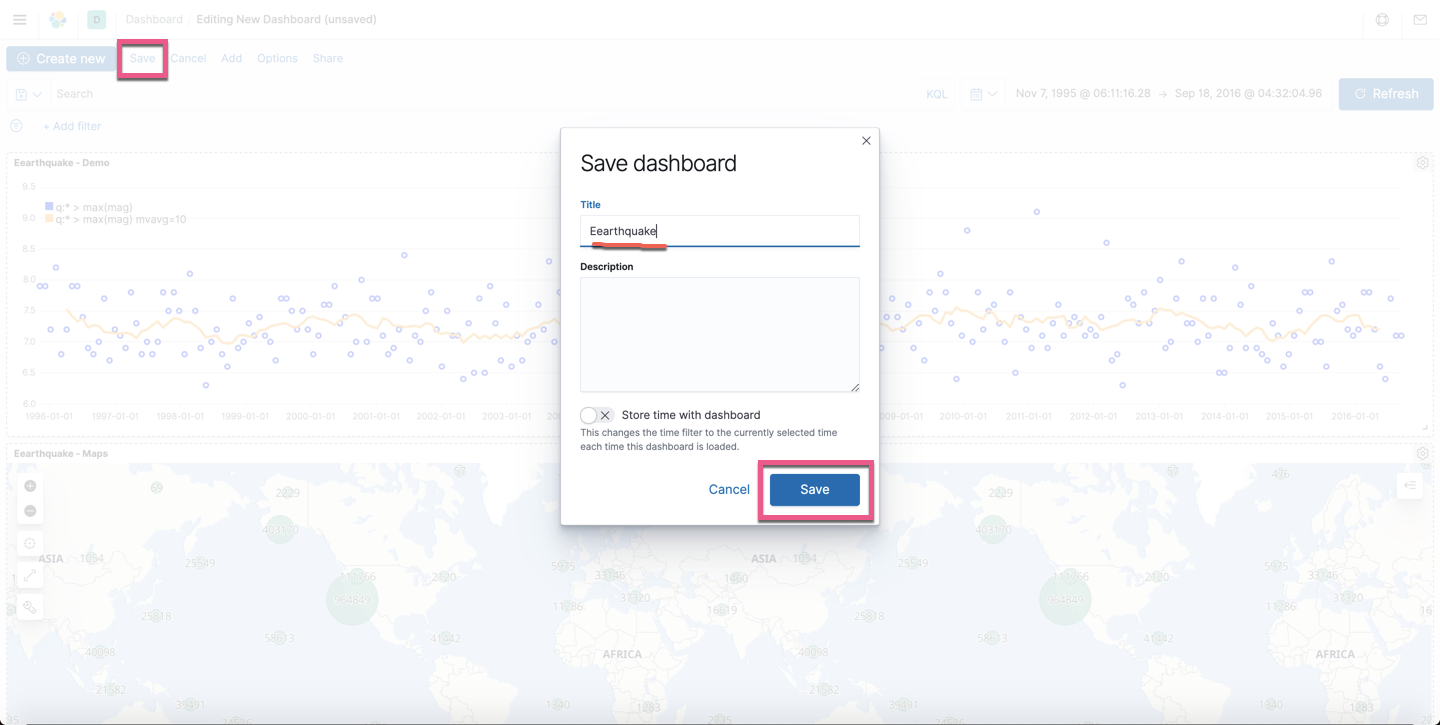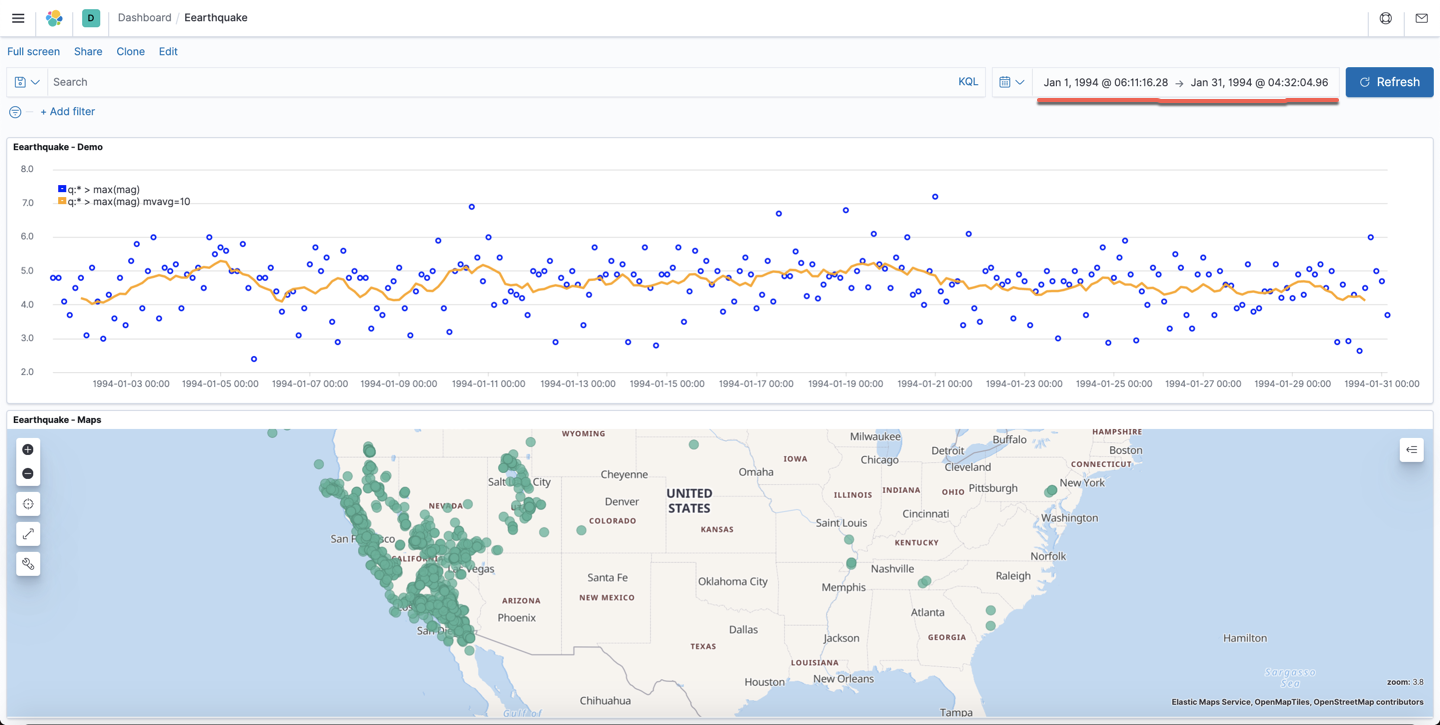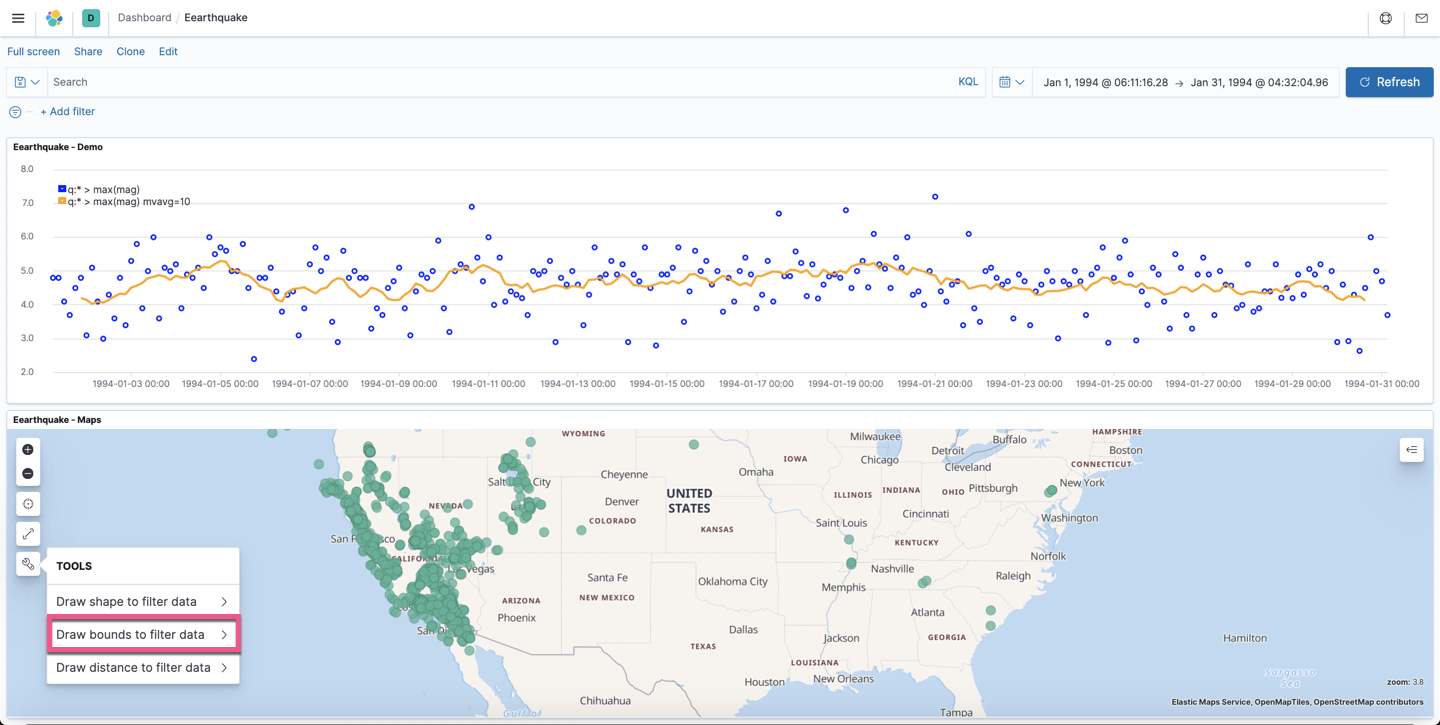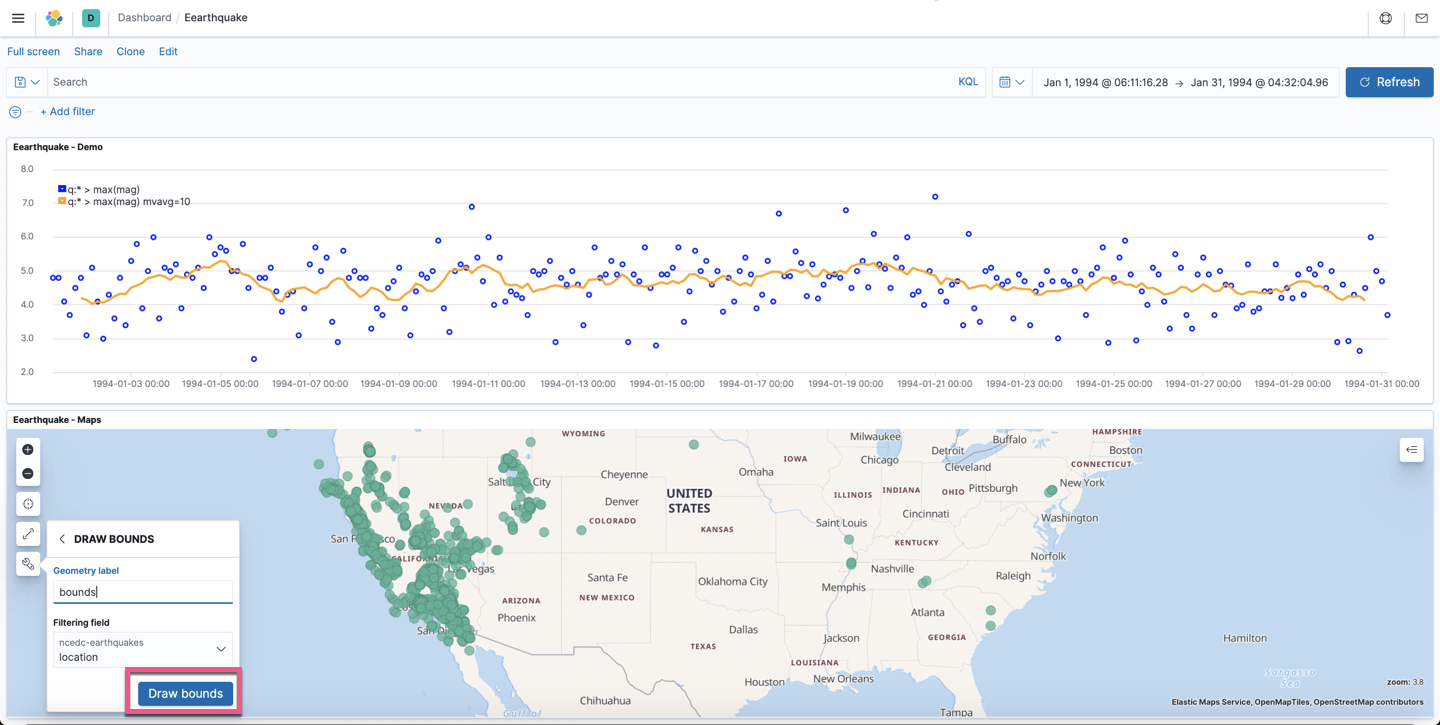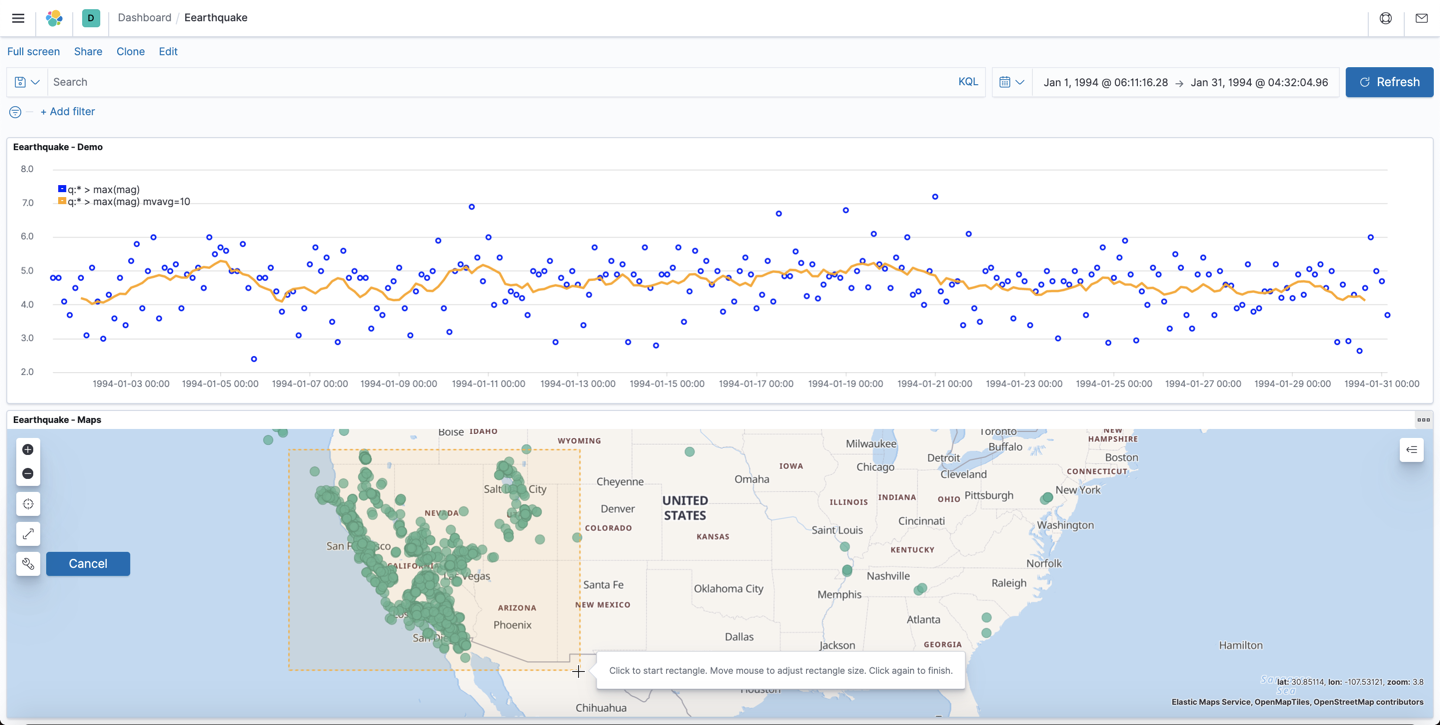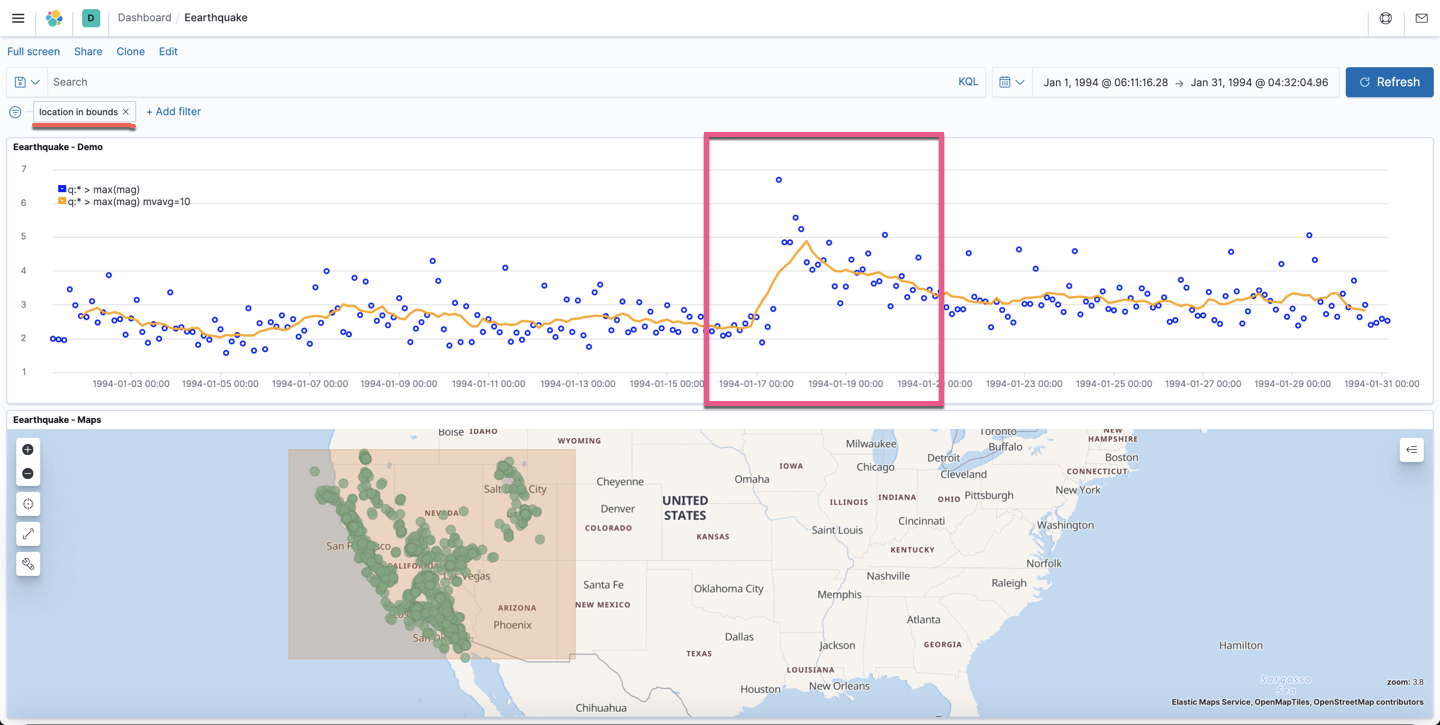10-282390
07-251992
02-252万+
01-065555
08-137160
08-087227
11-2564
11-2444
11-2348
11-1979